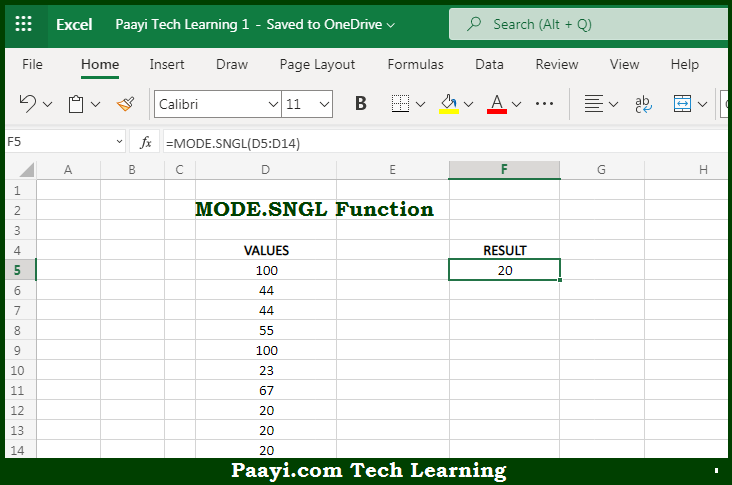# Learn How to Use Microsoft Excel MODE.SNGL Function

Written by | 0 Comments | 613 Views

In this article, you will learn how to use the Microsoft Excel MODE.SNGL function and its prime function in Microsoft Excel. You will also get to know the Microsoft Excel MODE.SNGL function return value and syntax with the help of some examples.

Microsoft Excel MODE.SNGL Function

The main purpose of the Microsoft Excel MODE.SNGL function is to return the most frequently occurring number. That implies, with the help of MODE.SNGL function you can able to return the most frequently occurring number in a numeric data set provided. Let's say, for example, =MODE.SNGL(1,2,4,4,4,4,5,5,5,6,6,6) will return 4. So, with the help of MODE.SNGL function, you can able to get the number that has occurred most of the time in the numeric data set.

Return Value of MODE.SNGL Function

The return value will be the number representing the mode.

Syntax of MODE.SNGL Function

=MODE.SNGL(number1, [number2], ...)

Where the arguments:

• number1: This is the number or cell reference that refers to numeric values.
• number2: This is the second number or cell reference that refers to numeric values (optional).

How to Use Microsoft Excel MODE.SNGL Function?So we know that, Microsoft Excel MODE.SNGL function you can able to return the most frequently occurring number. That implies, with the help of MODE.SNGL function you can able to return the most frequently occurring number in a numeric data set provided. Let's say, for example, =MODE.SNGL(1,2,4,4,4,4,5,5,5,6,6,6) will return 4. So, with the help of MODE.SNGL function, you can able to get the number that has occurred most of the time in the numeric data set.